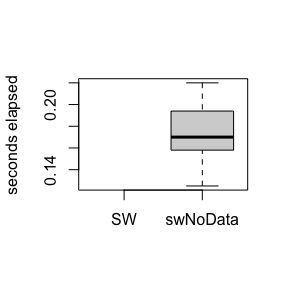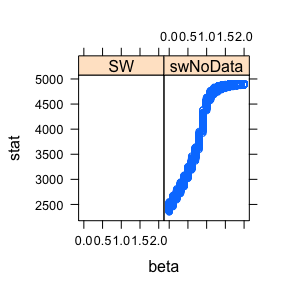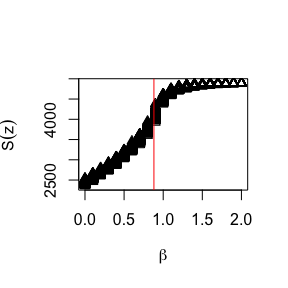swNoData

2021-04-11

Gibbs sampling was originally designed by Geman and Geman (1984) for drawing updates from the Gibbs distribution, hence the name. However, single-site Gibbs sampling exhibits poor mixing due to the posterior correlation between the pixel labels. Thus it is very slow to converge when the correlation (controlled by the inverse temperature $$\beta$$) is high.

The algorithm of Swendsen and Wang (1987) addresses this problem by forming clusters of neighbouring pixels, then updating all of the labels within a cluster to the same value. When simulating from the prior, such as a Potts model without an external field, this algorithm is very efficient.

The SW function in the PottsUtils package is implemented in a combination of R and C. The swNoData function in bayesImageS is implemented using RcppArmadillo, which gives it a speed advantage. It is worth noting that the intention of bayesImageS is not to replace PottsUtils. Rather, an efficient Swendsen-Wang algorithm is used as a building block for implementations of ABC (Grelaud et al. 2009), path sampling (Gelman and Meng 1998), and the exchange algorithm (Murray, Ghahramani, and MacKay 2006). These other algorithms will be covered in future posts.

There are two things that we want to keep track of in this simulation study: the speed of the algorithm and the distribution of the summary statistic. We will be using system.time(..) to measure both CPU and elapsed (wall clock) time taken for the same number of iterations, for a range of inverse temperatures:

beta <- seq(0,2,by=0.1)
tmMx.PU <- tmMx.bIS <- matrix(nrow=length(beta),ncol=2)
rownames(tmMx.PU) <- rownames(tmMx.bIS) <- beta
colnames(tmMx.PU) <- colnames(tmMx.bIS) <- c("user","elapsed")

We will discard the first 100 iterations as burn-in and keep the remaining 500.

iter <- 600
burn <- 100
samp.PU <- samp.bIS <- matrix(nrow=length(beta),ncol=iter-burn)

The distribution of pixel labels can be summarised by the sufficient statistic of the Potts model:

$S(z) = \sum_{i \sim \ell \in \mathscr{N}} \delta(z_i, z_\ell)$

where $$i \sim \ell \in \mathscr{N}$$ are all of the pairs of neighbours in the lattice (ie. the cliques) and $$\delta(u,v)$$ is 1 if $$u = v$$ and 0 otherwise (the Kronecker delta function). swNoData returns this automatically, but with SW we will need to use the function sufficientStat to calculate the sufficient statistic for the labels.

library(bayesImageS)

k <- 2
bcrit <- log(1 + sqrt(k))
maxSS <- nrow(edges)

for (i in 1:length(beta)) {
if (requireNamespace("PottsUtils", quietly = TRUE)) {
tm <- system.time(result <- PottsUtils::SW(iter,n,k,edges,beta=beta[i]))
tmMx.PU[i,"user"] <- tm["user.self"]
tmMx.PU[i,"elapsed"] <- tm["elapsed"]
res <- sufficientStat(result, neigh, block, k)
samp.PU[i,] <- res$sum[(burn+1):iter] print(paste("PottsUtils::SW",beta[i],tm["elapsed"],median(samp.PU[i,]))) } else { print("PottsUtils::SW unavailable on this platform.") } # bayesImageS tm <- system.time(result <- swNoData(beta[i],k,neigh,block,iter)) tmMx.bIS[i,"user"] <- tm["user.self"] tmMx.bIS[i,"elapsed"] <- tm["elapsed"] samp.bIS[i,] <- result$sum[(burn+1):iter]
print(paste("bayesImageS::swNoData",beta[i],tm["elapsed"],median(samp.bIS[i,])))
}
##  "PottsUtils::SW unavailable on this platform."
##  "bayesImageS::swNoData 0 0.125 2446"
##  "PottsUtils::SW unavailable on this platform."
##  "bayesImageS::swNoData 0.1 0.169999999999998 2571"
##  "PottsUtils::SW unavailable on this platform."
##  "bayesImageS::swNoData 0.2 0.187000000000001 2699.5"
##  "PottsUtils::SW unavailable on this platform."
##  "bayesImageS::swNoData 0.3 0.193999999999999 2834"
##  "PottsUtils::SW unavailable on this platform."
##  "bayesImageS::swNoData 0.4 0.216000000000001 2971"
##  "PottsUtils::SW unavailable on this platform."
##  "bayesImageS::swNoData 0.5 0.201000000000001 3137"
##  "PottsUtils::SW unavailable on this platform."
##  "bayesImageS::swNoData 0.6 0.212999999999997 3309.5"
##  "PottsUtils::SW unavailable on this platform."
##  "bayesImageS::swNoData 0.7 0.207000000000001 3519"
##  "PottsUtils::SW unavailable on this platform."
##  "bayesImageS::swNoData 0.8 0.220000000000002 3783"
##  "PottsUtils::SW unavailable on this platform."
##  "bayesImageS::swNoData 0.9 0.192999999999998 4183.5"
##  "PottsUtils::SW unavailable on this platform."
##  "bayesImageS::swNoData 1 0.173000000000002 4527"
##  "PottsUtils::SW unavailable on this platform."
##  "bayesImageS::swNoData 1.1 0.166 4676"
##  "PottsUtils::SW unavailable on this platform."
##  "bayesImageS::swNoData 1.2 0.167999999999999 4762"
##  "PottsUtils::SW unavailable on this platform."
##  "bayesImageS::swNoData 1.3 0.169 4813"
##  "PottsUtils::SW unavailable on this platform."
##  "bayesImageS::swNoData 1.4 0.155999999999999 4845"
##  "PottsUtils::SW unavailable on this platform."
##  "bayesImageS::swNoData 1.5 0.151999999999997 4863"
##  "PottsUtils::SW unavailable on this platform."
##  "bayesImageS::swNoData 1.6 0.164000000000001 4876"
##  "PottsUtils::SW unavailable on this platform."
##  "bayesImageS::swNoData 1.7 0.157999999999998 4883"
##  "PottsUtils::SW unavailable on this platform."
##  "bayesImageS::swNoData 1.8 0.148 4889"
##  "PottsUtils::SW unavailable on this platform."
##  "bayesImageS::swNoData 1.9 0.177000000000003 4893"
##  "PottsUtils::SW unavailable on this platform."
##  "bayesImageS::swNoData 2 0.157 4896"

Here is the comparison of elapsed times between the two algorithms (in seconds):

summary(tmMx.PU)
##    user         elapsed
##  Mode:logical   Mode:logical
##  NA's:21        NA's:21
summary(tmMx.bIS)
##       user           elapsed
##  Min.   :0.1180   Min.   :0.1250
##  1st Qu.:0.1480   1st Qu.:0.1580
##  Median :0.1610   Median :0.1700
##  Mean   :0.1663   Mean   :0.1769
##  3rd Qu.:0.1840   3rd Qu.:0.1940
##  Max.   :0.2030   Max.   :0.2200
boxplot(tmMx.PU[,"elapsed"],tmMx.bIS[,"elapsed"],ylab="seconds elapsed",names=c("SW","swNoData"))On average, swNoData using RcppArmadillo (Eddelbuettel and Sanderson 2014) is seven times faster than SW.

s_z <- c(samp.PU,samp.bIS)
s_x <- rep(beta,times=iter-burn)
s_a <- rep(1:2,each=length(beta)*(iter-burn))
s.frame <- data.frame(s_z,c(s_x,s_x),s_a)
names(s.frame) <- c("stat","beta","alg")
s.frame$alg <- factor(s_a,labels=c("SW","swNoData")) if (requireNamespace("lattice", quietly = TRUE)) { lattice::xyplot(stat ~ beta | alg, data=s.frame) }plot(c(s_x,s_x),s_z,pch=s_a,xlab=expression(beta),ylab=expression(S(z))) abline(v=bcrit,col="red")The overlap between the two distributions is almost complete, although it is a bit tricky to verify that statistically. The relationship between $$beta$$ and $$S(z)$$ is nonlinear and heteroskedastic. rowMeans(samp.bIS) - rowMeans(samp.PU) ##  NA NA NA NA NA NA NA NA NA NA NA NA NA NA NA NA NA NA NA NA NA apply(samp.PU, 1, sd) ##  NA NA NA NA NA NA NA NA NA NA NA NA NA NA NA NA NA NA NA NA NA apply(samp.bIS, 1, sd) ##  34.159419 34.260593 34.919729 35.856902 38.198227 41.902192 42.360515 ##  47.940617 54.571909 74.725912 44.237831 33.328516 24.102557 20.061483 ##  15.361047 12.389091 10.179723 8.006049 6.686276 5.617966 4.259688 s.frame$beta <- factor(c(s_x,s_x))
if (requireNamespace("PottsUtils", quietly = TRUE)) {
s.fit <- aov(stat ~ alg + beta, data=s.frame)
summary(s.fit)
TukeyHSD(s.fit,which="alg")
}